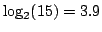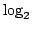Next: Summary of MI levels Up: content Previous: Calculating mutual information from

## Interpretations of mutual information

The MI value can be interpreted in a number of equivalent ways:

(i) The MI is the reduction in uncertainty about the stimulus after a response is observed. This is the standard information-theoretic interpretation. In the context studied here, without observing the response, the stimulus can be any one of thestimuli, resulting in an uncertainty that is quantified by the entropy of the set of stimuli, here equal tobits. If the mutual information between a neuron and the stimuli is e.g. 0.5 bit, observing the responses of the neuron reduces this entropy to 3.4. bits. Thus, the a-posteriori distribution over possible stimuli is less variable than the initial distribution. Observing more non-redundant neurons would reduce this uncertainty even more. If the uncertainty about the stimulus is, the stimulus is known with precision. Thus, theoretically, the responses oftotally non-redundant neurons, each with 0.5 bit/stimulus, are sufficient to fully specify the stimulus. I practice, neurons may be redundant and the actual number of neurons required to uniquely identify the stimulus may be substantially higher.

(ii) The MI is theof the number of different classes to which the stimuli can be subdivided after observing a response. This interpretation is tightly linked to the previous one, and is a concrete interpretation of the reduction in uncertainty.

(iii) The MI quantifies the differences between the responses to the various stimuli. The MI can be formally written as the the average divergence between the distribution of responses to a specific stimulus and the unconditional response distribution (superposition of the individual distributions to each of the specific stimuli). The divergence used here is the Kullback-Leibler (KL) divergence,. Thus, if the responses are independent of the stimulus, responses to any of the stimuli will be very similar (up to sampling issues) to the average distribution, and the KL distance will be small, resulting in a low MI. Conversely, when the responses strongly depend on the stimulus, the average response distribution is largely different from the response distribution to any specific stimulus, and as a result the KL distance is large and so is the MI.

In this view, the MI quantifies the stimulus effects on the responses. This view is closer to standard statistical tests such as 1-way ANOVA (that tests stimulus effects on the mean responses, assuming equal variance). However, standard statistical tests often have strong assumptions on the distributions of the responses, whereas the MI can be interpreted without any distributional assumptions.Next: Summary of MI levels Up: suppl_html Previous: Calculating mutual information from
Gal Chechik 2006-07-19